# 侧扫声呐图像小目标探测的数据降维方法Side-Scan Sonar Image Small Target Detection Method Based on Data Dimension Reduction

• 全文下载: PDF(712KB)    PP.72-79   DOI: 10.12677/AMS.2018.52009
• 下载量: 362  浏览量: 1,388   国家自然科学基金支持

With the continuous improvement of the resolution of Side Scan Sonar (SSS) image, its small target detection capability has gradually increased. For the problems of some of the traditional SSS image target detection methods need to provide sample images, and the methods based on statistical model need parameter estimation, which are supervision method, an unsupervised target detection method for SSS image is proposed. Firstly, the image is reduced in dimension by diffusion map; secondly, based on the data after dimension reduction, the target score is calculated by the target scoring equation which is defined by diffusion coordinate; finally, based on the target score, the target detection is achieved. The validity of the method was verified through the detection exper-iments of SSS images with different targets.

1. 引言

2. 扩散映射

$W\left({x}_{i},{x}_{j}\right)=\mathrm{exp}\left(-\frac{{‖{x}_{i}-{x}_{j}‖}^{2}}{{\delta }^{2}}\right)$ (1)

1) 数据点x的度

$d\left(x\right)=\underset{y\in X}{\sum }W\left(x,y\right)$ (2)

2) 转移概率

$P\left(x,y\right)=W\left(x,y\right)/d\left(x\right)$ (3)

$\underset{y\in X}{\sum }P\left(x,y\right)=1$ (4)

${P}_{t}\left(x,y\right)=\underset{l\ge 0}{\sum }{\lambda }_{l}^{t}{\phi }_{l}\left(x\right){\psi }_{l}\left(y\right)$ (5)

λ0为一常数，根据Mishne and Cohen  的研究，可进一步将扩散映射表示为： ${Y}_{t}:x\to {\left({\lambda }_{1}^{t}{\phi }_{\text{1}}\left(x\right),{\lambda }_{2}^{t}{\phi }_{\text{2}}\left(x\right),\cdots ,{\lambda }_{l}^{t}{\phi }_{l}\left(x\right)\right)}^{\text{T}}$

3) 扩散距离

${D}_{t}{\left(x,y\right)}^{2}=\underset{z\in X}{\sum }\frac{{\left({p}_{t}\left(x,z\right)-{p}_{t}\left(y,z\right)\right)}^{2}}{{\varphi }_{0}\left(z\right)}$ (6)

${D}_{t}^{2}\left(x,y\right)=\underset{j\ge 1}{\sum }{\lambda }_{j}^{2t}{\left({\phi }_{j}\left(x\right)-{\phi }_{j}\left(y\right)\right)}^{2}={‖\left(Y\left(x\right)-Y\left(y\right)\right)‖}^{2}$ (7)

3. 基于扩散映射的目标探测

$c=1-\mathrm{exp}\left\{-\frac{1}{k}\underset{t=1}{\overset{k}{\sum }}\frac{D\left({p}_{i},{p}_{j}\right)/2{\sigma }_{k}}{1+3×{d}_{\text{position}}\left({p}_{i},{p}_{j}\right)}\right\}$ (8)

4. 实验及分析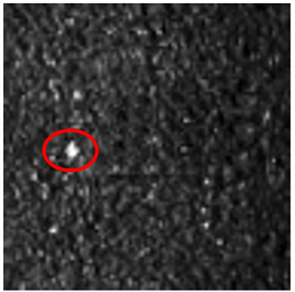Figure 1. Side scan sonar image with single distinct target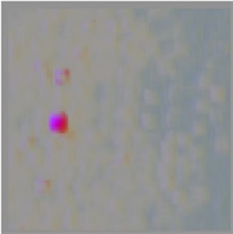(a)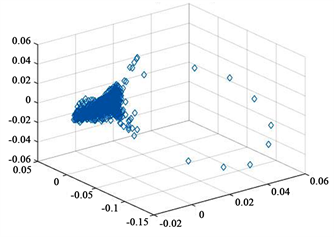(b)

Figure 2. The first three-dimensional data of the diffusion coordinates, (a) color image; (b) distribution of the coordinate data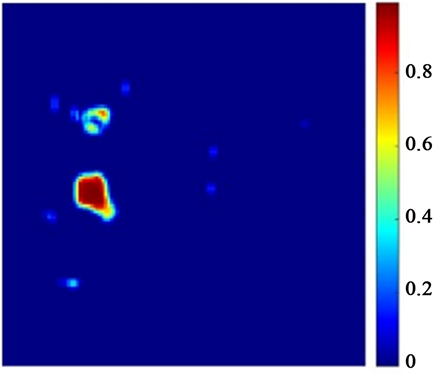Figure 3. The target scoring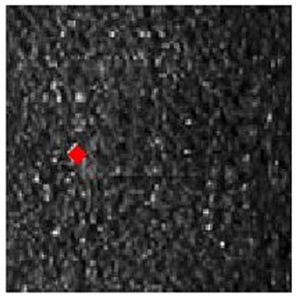Figure 4. The target detection result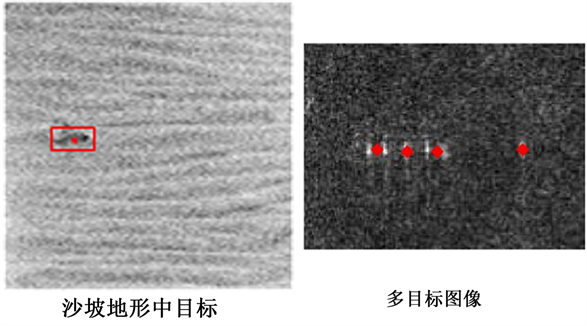Figure 5. Side scan sonar image target detection results with Multi-target or target in sand slope topography

5. 讨论

6. 结论及建议

1) 基于扩散映射进行数据降维，有效解决了侧扫声呐图像数据量大，Matlab计算大型矩阵效率低甚至无法进行运算的问题；

2) 利用扩散映射降维之后的扩散坐标，基于扩散距离利用目标得分方程可实现侧扫声呐图像中小目标的准确探测，且无需样本图像；

3) 目标得分方程定义方式，对目标探测结果影响很大，顾及海底复杂纹理背景差异，后续需深入研究联合图像纹理特征的目标得分方程定义公式。

NOTES

*通讯作者。

  Blondel, P. (2009) The Handbook of Side Scan Sonar. Springer, UK. https://doi.org/10.1007/978-3-540-49886-5  Davy, C.M. and Fenton, M.B. (2013) Technical Note: Side-Scan Sonar Enables Rapid Detection of Aquatic Reptiles in Turbid Lotic Systems. European Journal of Wildlife Research, 59, 123-127. https://doi.org/10.1007/s10344-012-0680-9  Powers, J., Brewer, S.K., Long, J.M. and Campbell, T. (2015) Evaluating the Use of Side-Scan Sonar for Detecting Freshwater Mussel Beds in Turbid River Environments. Hydrobiologia, 743, 127-137. https://doi.org/10.1007/s10750-014-2017-z  Fakiris, E., Papatheodorou, G., Geraga, M. and Ferentinos, G. (2016) An Automatic Target Detection Algorithm for Swath Sonar Backscatter Imagery, Using Image Texture and Independent Component Analysis. Remote Sensing, 8, 1-13. https://doi.org/10.3390/rs8050373  Dobeck, G.J. and Hyland, J.C. (1997) Automated Detection and Classification of Sea Mines in Sonar Imagery. AeroSense, International Society for Optics and Photonics, Panama City, 90-110.  Dura, E., Zhang, Y., Liao, X., Dobeck, G.J. and Carin, L. (2005) Active Learning for Detection of Mine-Like Objects in Side-Scan Sonar Imagery. IEEE Journal of Oceanic Engineering, 30, 360-371. https://doi.org/10.1109/JOE.2005.850931  Grasso, R. and Spina, F. (2006) Small Bottom Object Density Analysis from Side Scan Sonar Data by a Mathematical Morphology Detector. 9th IEEE International Conference in Information Fusion, Florence, 10-13 July 2006, 1-8. https://doi.org/10.1109/ICIF.2006.301753  田晓东, 刘忠. 基于灰度分布模型的声呐图像目标检测算法[J]. 系统工程与电子技术, 2007, 29(5): 695-698.  梁旭姣, 程永强, 周超, 等. 基于灰度分布信息的鲟鱼声呐图像分析研究[J]. 渔业现代化, 2014, 41(6): 32-35.  李娟娟, 马硕, 朱枫, 等. 基于主动轮廓的声呐图像水雷识别方法[J]. 计算机应用研究, 2014, 31(12): 3841-3844.  赵小红. 基于Duffing方程的强混响下弱信号检测[D]: [硕士学位论文]. 哈尔滨: 哈尔滨工程大学, 2012.  库安邦, 周兴华, 李冠泽. 不同型号侧扫声呐分辨能力和扫宽能力的比较分析[J]. 测绘地理信息, 2018(1): 1-5.  klein公司网站[EB/OL]. http://www.l-3mps.com/Klein/sidescansonar.aspx  KongsBerg公司网站[EB/OL]. http://www.km.kongsberg.com/  魏莱, 王守觉. 基于流形距离的半监督判别分析[J]. 软件学报, 2010, 21(10): 2445-2453.  Rabin, N. and Coifman, R.R. (2012) Heterogeneous Datasets Representation and Learning Using Diffusion Maps and Laplacian Pyramids. In SDM, 189-199.  Mishne, G. and Cohen, I. (2013) Multiscale Anomaly Detection Using Diffusion Maps. IEEE Journal of Selected Topics in Signal Processing, 7, 111-123. https://doi.org/10.1109/JSTSP.2012.2232279  Mishne, G. and Cohen, I. (2014) Multiscale Anomaly Detection Using Diffusion Maps and Saliency Score. IEEE International Conference in Acoustics, Speech and Signal Processing (ICASSP), Florence, 4-9 May 2014, 2823-2827. https://doi.org/10.1109/ICASSP.2014.6854115